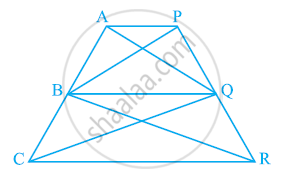Share

# In the given figure, AP || BQ || CR. Prove that ar (AQC) = ar (PBR). - CBSE Class 9 - Mathematics

ConceptTriangles on the Same Base and Between the Same Parallels

#### Question

In the given figure, AP || BQ || CR. Prove that ar (AQC) = ar (PBR).#### Solution

Since ΔABQ and ΔPBQ lie on the same base BQ and are between the same parallels AP and BQ,

∴ Area (ΔABQ) = Area (ΔPBQ) ... (1)

Again, ΔBCQ and ΔBRQ lie on the same base BQ and are between the same parallels BQ and CR.

∴ Area (ΔBCQ) = Area (ΔBRQ) ... (2)

On adding equations (1) and (2), we obtain

Area (ΔABQ) + Area (ΔBCQ) = Area (ΔPBQ) + Area (ΔBRQ)

⇒ Area (ΔAQC) = Area (ΔPBR)

Is there an error in this question or solution?

#### APPEARS IN

NCERT Solution for Mathematics Class 9 (2018 to Current)
Chapter 9: Areas of Parallelograms and Triangles
Ex. 9.30 | Q: 14 | Page no. 164

#### Video TutorialsVIEW ALL 

Solution In the given figure, AP || BQ || CR. Prove that ar (AQC) = ar (PBR). Concept: Triangles on the Same Base and Between the Same Parallels.
S